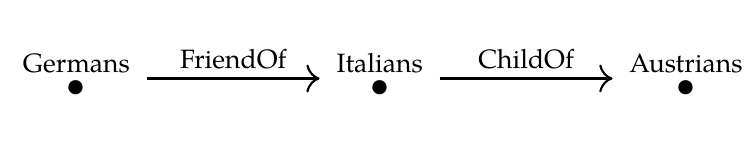We've seen that a database schema is just a category \$$\mathcal{C}\$$, and a database built on that schema is just a functor \$$F: \mathcal{C} \to \textbf{Set}\$$. What can we do with this realization? A lot. First, we can try replacing \$$\textbf{Set}\$$ with some other category, and see what happens! We get some useful variants. [Matthew Doty](https://forum.azimuthproject.org/discussion/comment/19050/#Comment_19050) came up with a nice one last time, and I'd like to expand on it and set it in a larger context. The category \$$\textbf{Set}\$$ has sets as object and _functions_ as morphisms. But functions are just a special case of _relations_. **Definition.** Given sets \$$X\$$ and \$$Y\$$, a **relation** from \$$X\$$ to \$$Y\$$ is a subset of \$$X \times Y\$$. For example we could have a relation \$$\textrm{FriendOf}\$$ from the set \$$X\$$ of Germans to the set \$$Y\$$ of Italians. Then \$$(x,y) \in \textrm{FriendOf}\$$ means that \$$x\$$ is a German, \$$y\$$ is an Italian, and \$$x\$$ is a friend of \$$y\$$. When \$$R\$$ is a relation from \$$X\$$ to \$$Y\$$, people often write \$$R : X \nrightarrow Y\$$. This looks like the notation for functions, but also different, so we don't get mixed up. There's a good reason for this. Any function \$$f : X \to Y\$$ gives a relation from \$$X\$$ to \$$Y\$$, namely the subset $\\{ (x, f(x)) : \; x \in X \\} \subseteq X \times Y .$ Indeed lots of people these days _define_ a function from \$$X\$$ to \$$Y\$$ to be a special kind of relation \$$R: X \nrightarrow Y\$$, namely one that is: 1. **Deterministic:** for any \$$x \in X\$$ there is at most one \$$y \in Y\$$ with \$$(x,y) \in R\$$. 2. **Total:** for any \$$x \in X\$$ there is at least one \$$y \in Y\$$ with \$$(x,y) \in R\$$. We can compose relations in a way that generalizes how we compose functions: **Definition.** Given relations \$$R : X \to Y\$$ and \$$S : Y \to Z\$$, define the **composite** relation \$$S \circ R : X \to Z \$$ by $S \circ R = \\{ (x,z) : \; \textrm{ there exists } y \in Y \textrm{ such that } (x,y) \in R \textrm{ and } (y,z) \in S \\}$ For example, if we compose the relation $\textrm{FriendOf} : \\{\textrm{Germans}\\} \nrightarrow \\{ \textrm{Italians}\\}$ and the relation $\textrm{ChildOf} : \\{\textrm{Italians}\\} \nrightarrow \\{ \textrm{Austrians}\\}$ we get a relation $\textrm{ChildOf} \circ \textrm{FriendOf}: \\{\textrm{Germans}\\} \nrightarrow \\{ \textrm{Austrians}\\}$ where \$$(x,z) \$$ is in this relation if \$$x\$$ is a German, \$$y\$$ is an Austrian, and \$$x\$$ is a friend of a child of \$$z\$$. The word "relation" is not accidental: in every culture, people have always spent a lot of time keeping track of who is related to whom, and in what way! So, the following category is built deep into our brains: **Puzzle 114.** Show that there is a category \$$\textbf{Rel}\$$ where: 1. objects are sets; 2. a morphism \$$R : X \nrightarrow Y\$$ is a relation from \$$X\$$ to \$$Y\$$; 3. relations are composed as described above; 4. the identity relation \$$1_X : X \nrightarrow X \$$ is $1_X = \\{ (x,x) : x \in X \\} .$ What do you need to do, to show this? You need to check the [laws in the definition of a category](https://forum.azimuthproject.org/discussion/2198/lecture-34-chapter-3-categories/p1). If you don't remember those, punish yourself and then go look them up! **Puzzle 115.** There is an obvious functor \$$i: \textbf{Set} \to \textbf{Rel}\$$. What is it, why is it a functor, and why do people say this functor makes \$$\textbf{Set}\$$ into a [subcategory](https://en.wikipedia.org/wiki/Subcategory) of \$$\textbf{Rel}\$$? Using this idea we can imagine a new kind of database! We'll take our database schema to be a category \$$\mathcal{C}\$$ as before, but now we'll define a database built using this schema to be a functor $F : \mathcal{C} \to \mathbf{Rel} .$ Now \$$F\$$ maps each object of \$$\mathcal{C}\$$ to a set as before, but it maps each morphism to a _relation_ between sets! For example, we could take \$$\mathcal{C}\$$ to be the free category on this graph:Then a database will be a functor \$$F : \mathcal{C} \to \mathbf{Rel} \$$ that picks out a set of Germans, a set of Italians, a set of Austrians, a _relation_ from Germans to Italians, and a _relation_ from Italians to Austrians. This is good! If we used a functor \$$F : \mathcal{C} \to \mathbf{Set} \$$, we'd be forcing each German to be the friend of _exactly one_ Italian, and each Italian to be the child of _exactly one_ Austrian! That's because functions are relations that are both deterministic and total. So, we get more flexibility in our theory of databases if we let other categories take the place of \$$\textbf{Set}\$$. I'll conclude with some puzzles that explore another example. **Definition.** Given sets \$$X\$$ and \$$Y\$$, a **[partial function](https://en.wikipedia.org/wiki/Partial_function)** \$$f : X \nrightarrow Y\$$ is a relation from \$$X\$$ to \$$Y\$$ that is deterministic but not necessarily total. **Puzzle 116.** Show that if we compose two relations that are partial functions, the result is a partial function. Using this we get a category \$$\textbf{Par}\$$ with 1. sets as objects, 2. partial functions as morphisms, 3. composition as just described, 4. the usual identity relations as identity morphisms. Every function is a partial function, and every partial function is a relation, but not conversely! In fact \$$\mathbf{Set} \$$ is a subcategory of \$$\mathbf{Par}\$$, and \$$\mathbf{Par}\$$ is a subcategory of \$$\mathbf{Rel}\$$. (I know I haven't said what a [subcategory](https://en.wikipedia.org/wiki/Subcategory#Formal_definition) is, but you can look it up.) **Puzzle 117.** Start with a graph \$$G\$$ having one node \$$\textrm{Person}\$$ and one edge \$$\textrm{BestFriend}\$$ from that node to itself. This gives a free category \$$\mathcal{C} = \mathbf{Free}(G)\$$. How many functors \$$F : \mathcal{C} \to \mathbf{Par}\$$ are possible if $F(\textrm{Person}) = \\{ \textrm{Anna}, \textrm{Jordan}, \textrm{Lisa}, \textrm{Ryan} \\} ?$ What sort of situations do these functors describe? How is this different from [Puzzle 111](https://forum.azimuthproject.org/discussion/2220/lecture-39-chapter-3-databases/p1)? **Puzzle 118.** How many functors \$$F : \mathcal{C} \to \mathbf{Rel} \$$ are there? What situations do these describe? **[To read other lectures go here.](http://www.azimuthproject.org/azimuth/show/Applied+Category+Theory#Chapter_3)**